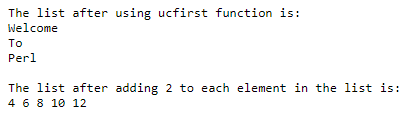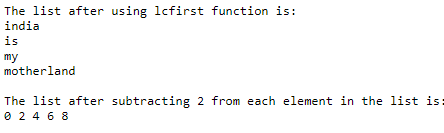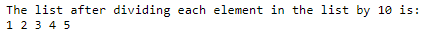# Perl map | Working of map() function in Perl with examples

33## Introduction to Perl map

Whenever we are working with a list in Perl, if we want to evaluate the expression or block for every element in the list, we make use of a function called the map() function in Perl. The symbol \$_ holds the current element’s value during each iteration of the list. And map() function can execute an expression on every element present in the array and return a new array consisting of resulting elements after the execution of the expression. This map() function is usually a one-to-one transformation of the original list to the resulting list, but the resulting list can be shorter or longer than the original list.

The syntax to declare map() function in Perl is as follows:

```map(EXPR, List); map(BLOCK, List);```

where EXPR is the expression to be evaluated on each element of the list specified by the name List and

BLOCK is the block to be evaluated on each element of the list specified by the name List.

### Working of map() function in Perl

• Whenever we are working with a list in Perl, if we want to evaluate the expression or block for every element in the list, we make use of a function called the map() function in Perl.
• The symbol \$_ holds the current element’s value during each iteration of the list.
• The map() function can execute an expression on every element present in the array and return a new array consisting of resulting elements after the execution of the expression.
• The map() function is usually a one-to-one transformation of the original list to the resulting list, but the resulting list can be shorter or longer than the original list.

### Examples of Perl map

Here are the following examples mention below

#### Example #1

Perl program to illustrate the working of map() function to display each element in the given list beginning with a capital letter and to perform an operation on each element in the given list and display the resulting list:

Code:

```#a list consisting of elements is stored in a variable called firstlist @firstlist = ('welcome', 'to', 'perl'); #map() function is used to display each element of the firstlist beginning with capital letter @resultlist = map(ucfirst, @firstlist); print "The list after using ucfirst function is:n"; #iterating through the resultinglist to display each element in the list one after the other foreach \$element ( @resultlist ) { print "\$elementn"; } print "n"; #a list of numbers is stored in a variable called noslist @noslist = (2, 4, 6, 8, 10); #map() function is used to add 2 to each element in the list @resultnoslist = map{\$_ + 2} @noslist; print "The list after adding 2 to each element in the list is:n"; #the resulting list is displayed as the output on the screen print "@resultnoslistn";```

Output:In the above program, a list consisting of elements is stored in a variable called firstlist. Then map() function is used to display each element of the firstlist beginning with a capital letter. Then the resulting list is iterated to display each element in the list one after the other. Then a list of numbers is stored in a variable called noslist. Then map() function is used to add 2 to each element in the list. Then the resulting list is displayed as the output on the screen.

#### Example #2

Perl program to illustrate the working of map() function to display each element in the given list beginning with a small letter and to perform an operation on each element in the given list and display the resulting list:

Code:

```#a list consisting of elements is stored in a variable called firstlist @firstlist = ('India', 'Is', 'My', 'Motherland'); #map() function is used to display each element of the firstlist beginning with small letter @resultlist = map(lcfirst, @firstlist); print "The list after using lcfirst function is:n"; #iterating through the resultinglist to display each element in the list one after the other foreach \$element ( @resultlist ) { print "\$elementn"; } print "n"; #a list of numbers is stored in a variable called noslist @noslist = (2, 4, 6, 8, 10); #map() function is used to subtract 2 to from each element in the list @resultnoslist = map{\$_ - 2} @noslist; print "The list after subtracting 2 from each element in the list is:n"; #the resulting list is displayed as the output on the screen print "@resultnoslistn";```

Output:In the above program, a list consisting of elements is stored in a variable called firstlist. Then map() function is used to display each element of the firstlist, beginning with a small letter. Then the resulting list is iterated to display each element in the list one after the other. Then a list of numbers is stored in a variable called noslist. Then map() function is used to subtract 2 from each element in the list. Then the resulting list is displayed as the output on the screen.

#### Example #3

Perl program to illustrate the working of the map() function to perform an operation on each element in the given list and display the resulting list:

Code:

```#a list of numbers is stored in a variable called noslist @noslist = (10, 20, 30, 40, 50); #map() function is used to divide each element in the list by 10 @resultnoslist = map{\$_ /10} @noslist; print "The list after dividing each element in the list by 10 is:n"; #the resulting list is displayed as the output on the screen print "@resultnoslistn";```

Output:In the above program, a list of numbers is stored in a variable called noslist. Then map() function is used to divide each element in the list by 10. Then the resulting list is displayed as the output on the screen.

### Recommended Articles

This is a guide to the Perl map. Here we have discussed the concept of map() function in Perl through definition, syntax, and working of map() function in Perl through programming examples and their outputs. You may also have a look at the following articles to learn more –

The post Perl map appeared first on EDUCBA.